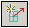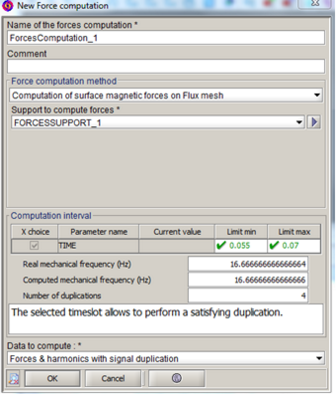# Step 2 : Computation and export of the forces

## Introduction

In this part, the method of creating the computation of surface magnetic forces on Flux mesh is described.

It is available through the menu Force computation > New or through the icon## Creation of force computation

The steps of the computation creation are described below. Images of the creation box of the computation are also available.

Step Action
1 Choose the name of the computation (+comment)
2 Choose the computation method Computation of surface magnetic forces on Flux mesh
3 Choose the computation support created beforehand
4

In magnetic transient:

Choose the computation interval according to the choice of applying or not a temporal duplication in the field Data to compute (See Spatial and temporal definition ).

Reminders :

• the initial step and the final step are repeated
• Do not consider the first step of the scenario as the first step of the computation

Choose the forces to compute:

• Computing continuous values of forces
• Computing instantaneous values of forces
5

In magnetic transient:

Choose the data to compute. It is about choosing :

• The application, or not, of a temporal duplication (choice connected with stage 4)
• The choice to compute:
• Only the forces
• Or the forces with their harmonics

It is strongly advisable to calculate the harmonics (if it has a physical meaning), as the computation of the harmonics is rapid.

6

In magnetic transient:

In case of temporal duplication, verify if the time interval is in good correlation with :

• The real fundamental frequency
• The computed fundamental frequency based on the computation interval
• The number of duplications
• the number of computed harmonics (see Spatial and temporal definition last paragraph for more information)

See Tools of geometric reconstruction and temporal duplication .

7 Validate by OK
8 Visualize the arrows of the forces or of the harmonics (See Postprocessing with forces arrows)

Step 1 to 7: